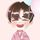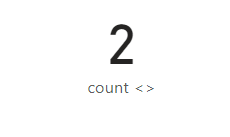cancel
Showing results for
Did you mean:Helper I

## Need Help Please. I need to COUNT a value with an OR and an AND condition from another table.

I need a COUNT of the IDs in Worksheet A that match the following criteria:

Column A is greater than 25 OR Column B greater than 250

AND

The ID from Worksheet A equals an ID2 from Worksheet B

The COUNT should be 17.

Here is the link for the Power BI file.  Thanks!!!

1 ACCEPTED SOLUTIONSuper User

Hi,

``````expected result measure: =
COUNTROWS (
CALCULATETABLE (
DISTINCT ( Worksheet_A[ID] ),
FILTER (
Worksheet_A,
OR ( Worksheet_A[A] > 25, Worksheet_A[B] > 250 )
&& Worksheet_A[ID] IN DISTINCT ( Worksheet_B[ID2] )
)
)
)
``````

If this post helps, then please consider accepting it as the solution to help other members find it faster, and give a big thumbs up.

6 REPLIES 6Community Support

Hi @The_Clam ,

Actually in my test ,the condition1 returns 19 rows not 17 and the condition2 will return 2 rows.

You may firstly refer to @Jihwan_Kim 's method which  works fine.

Or my method is:

``````count <> =
var _t= DISTINCT(SUMMARIZE(FILTER('Worksheet_A',[A]>25 || [B]>250),[ID]))
RETURN COUNTROWS(FILTER(_t,( [ID] IN VALUES(Worksheet_B[ID2])) =FALSE()  ))``````Best Regards,
Eyelyn Qin
If this post helps, then please consider Accept it as the solution to help the other members find it more quickly.Super User

Hi,

Here's what i would do.

Write this calculated column formula in worksheet_A

``Available in worksheet_B = LOOKUPVALUE(Worksheet_B[ID2],Worksheet_B[ID2],Worksheet_A[ID])``

Write this measure and drag it to a card visual

``Measure = calculate(distinctcount(Worksheet_A[ID]),FILTER(Worksheet_A,Worksheet_A[ID]=Worksheet_A[Available in worksheet_B]&&(Worksheet_A[A]>25||Worksheet_A[B]>250)))``

The result will be 19.

Hope this helps.

Regards,
Ashish Mathur
http://www.ashishmathur.comSuper UserFor fun only, see the power of Excel worksheet formula,Thanks to the great efforts by MS engineers to simplify syntax of DAX! Most beginners are SUCCESSFULLY MISLED to think that they could easily master DAX; but it turns out that the intricacy of the most frequently used RANKX() is still way beyond their comprehension! DAX is simple, but NOT EASY!Helper I

@Jihwan_Kim, Yes!  That works.  What if I wanted to do the opposite for the 2nd criteria, so it would be....."AND The ID from Worksheet A does NOT equal an ID2 from Worksheet B".  What is the opposite of IN?  I tried putting NOT IN, but it didn't work.Super User

Hi,

``````expected result measure: =
COUNTROWS (
CALCULATETABLE (
DISTINCT ( Worksheet_A[ID] ),
FILTER (
Worksheet_A,
OR ( Worksheet_A[A] > 25, Worksheet_A[B] > 250 )
&& NOT( Worksheet_A[ID] IN DISTINCT ( Worksheet_B[ID2] ) )
)
)
)``````

If this post helps, then please consider accepting it as the solution to help other members find it faster, and give a big thumbs up.Super User

Hi,

``````expected result measure: =
COUNTROWS (
CALCULATETABLE (
DISTINCT ( Worksheet_A[ID] ),
FILTER (
Worksheet_A,
OR ( Worksheet_A[A] > 25, Worksheet_A[B] > 250 )
&& Worksheet_A[ID] IN DISTINCT ( Worksheet_B[ID2] )
)
)
)
``````

If this post helps, then please consider accepting it as the solution to help other members find it faster, and give a big thumbs up.Announcements#### Exclusive opportunity for Women!

Join us for a free, hands-on Microsoft workshop led by women trainers for women where you will learn how to build a Dashboard in a Day!#### Power Platform Conference-Power BI and Fabric Sessions

Join us Oct 1 - 6 in Las Vegas for the Microsoft Power Platform Conference.Top Solution Authors
Top Kudoed Authors
Users online (1,862)